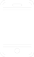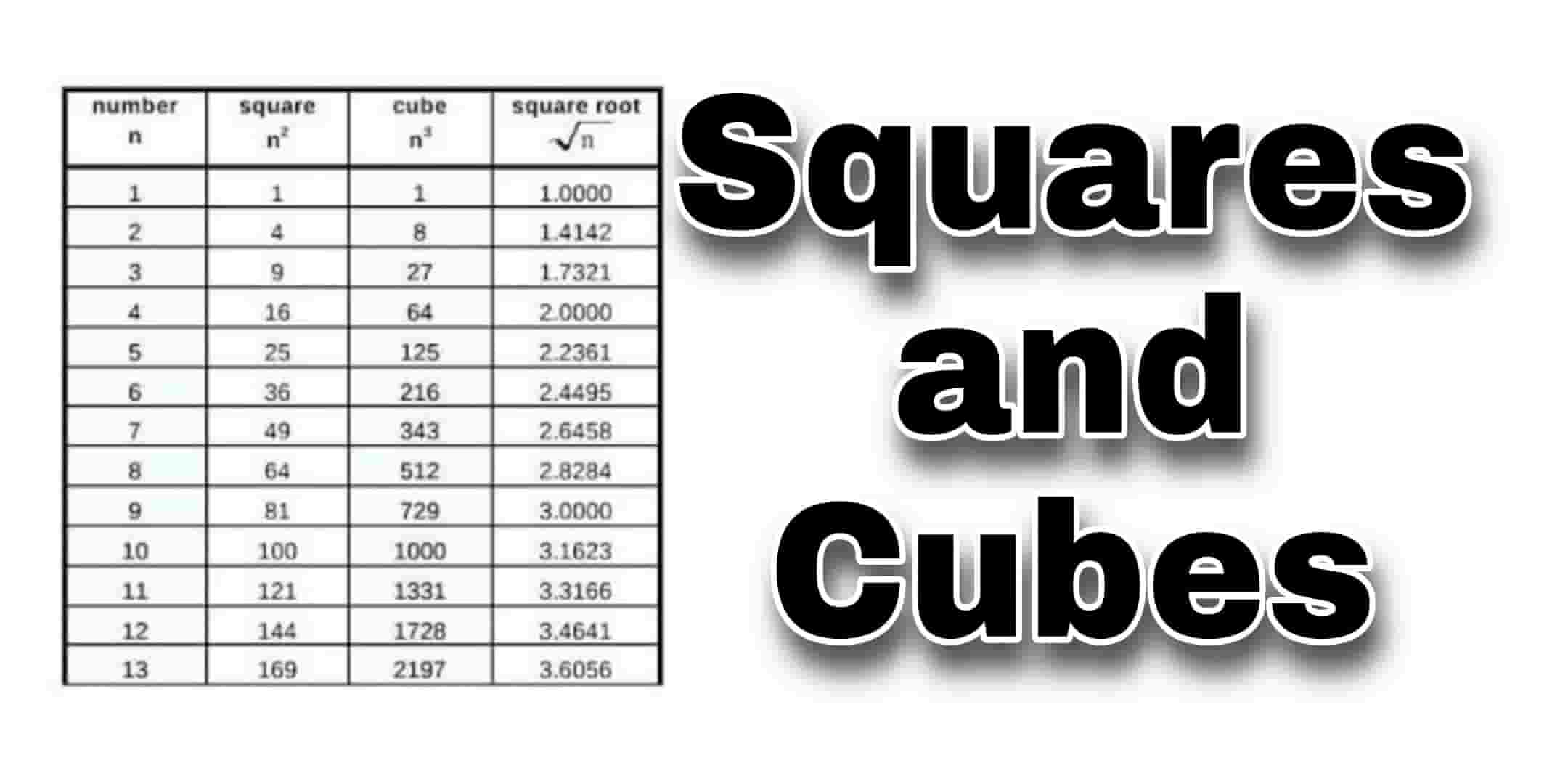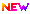# Squares and Cubes For Defence and Paramilitary JobsSquares and Cubes For Defence and Paramilitary Jobs Squares and cubes form an important part of Quantitative Aptitude section. Basically, these are also important from all upcoming exams’ point of view. Knowing squares and roots is quite important for an aspirant to know in order to enhance their calculation speed especially in simplification & approximation questions. Such questions involving squares and cubes can always be asked in Army All Trades, CISF,BSF,CRPF and also in Navy,Airforce any exams so in this article we are explaining the aspirants know the squares numbers from 1 to 40 and cubes of numbers from 1 to 30 Minimun.10th base Defence JobsITI based Defence JobsDiploma base Defence JobsInter Base Defence Jobs

## Squares and Cubes For Defence and Paramilitary Jobs

What is a Square:

a square number or perfect square is an integer that is the square of an integer.in other words, it is the product of some integer with itself. For example, 9 is a square number, since it can be written as 3 × 3.The usual notation for the square of a number n is not the product n × n, but the equivalent exponentiation n2, usually pronounced as “n squared”.

What is a Cube:

the cube of a number n is its third power, that is, the result of multiplying three instances of n together. The cube of a number or any other mathematical expression is denoted by a superscript 3, n3 = n × n2 = n × n × n.

General Knowlwdge Questions Part-I

General Knowlwdge Questions Part-II

General Knowlwdge Questions Part-III

General Science Previous Questions